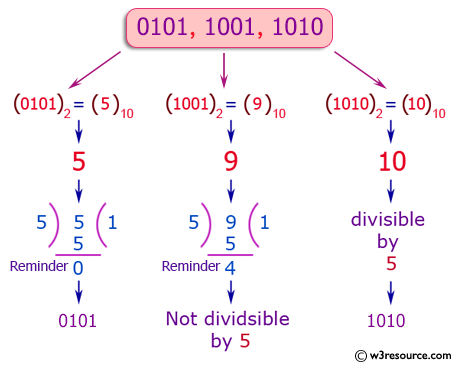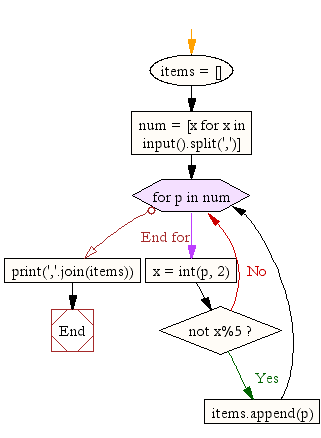﻿ Python Exercise: Print the 4 digit binary numbers that are divisible by 5 - w3resource# Python Exercise: Print the 4 digit binary numbers that are divisible by 5

## Python Conditional: Exercise-13 with Solution

Write a Python program which accepts a sequence of comma separated 4 digit binary numbers as its input and print the numbers that are divisible by 5 in a comma separated sequence.

Pictorial Presentation:Sample Solution:

Python Code:

``````items = []
num = [x for x in input().split(',')]
for p in num:
x = int(p, 2)
if not x%5:
items.append(p)
print(','.join(items))
```
```

Sample Output:

```0001,0010,0011,0100,0101,0110,0111
0101
```

Flowchart:## Visualize Python code execution:

The following tool visualize what the computer is doing step-by-step as it executes the said program:

Python Code Editor:

Have another way to solve this solution? Contribute your code (and comments) through Disqus.

What is the difficulty level of this exercise?

Test your Python skills with w3resource's quiz

﻿

## Python: Tips of the Day

Returns a list with n elements removed from the beginning

Example:

```def tips_take(itr, n = 1):
return itr[:n]
print(tips_take([1, 2, 3], 5))
print(tips_take([1, 2, 3], 0))
```

Output:

```[1, 2, 3]
[]
```